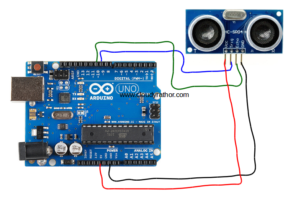# How to program the Ultrasonic Sensor(HC-SR04)

### How to program the Ultrasonic Sensor(HC-SR04)

One of the most amazing sensor to which i deal with is Ultrasonic sensor, the reason is, it uses the speed of sound to detect the distance so lets program it.

Before going to the programming we need to know the basic of Ultrasonic sensor. So this is a device which calculate the distance of an obstacle or object by using sound waves. It send the sound of specific frequency to the object and hear that sound, The time taken by the sound to go and come back is calculated to find out the distance.

We learn the concept of sound at school and we know that sound travels through air at the speed of 344 meters or we can say 1129 feet. So if we send the sound to one direction it need to travel back so that sensor can detect it. But some time some objects not detected by the ultrasonic sensor because of its shape and the sound wave is redirected to the other direction.

So to program the sensor we know the speed of sound that is 344 meters/second and formula for distance is

Distance =  Speed * Time but we have a round trip of sound so divide it by 2

Distance = (Speed of Sound * Time Taken)/2

To use this sensor you need to use Trig pin on High which will generate the ultrasound which will go forward and the echo will be received by the echo pin. Now this is the job of echo pin to get the difference and our program will give the output in the form of Distance.// defines pins numbers

const int trigPin = 9; //trigger pin for sending the sound to the object

const int echoPin = 10; // It will receive the sound for further calculation

// defines variables

long duration;

int distance;

void setup() {

pinMode(trigPin, OUTPUT);    // Sets the trigPin as an Output

pinMode(echoPin, INPUT);    // Sets the echoPin as an Input

Serial.begin(9600); // Starts the serial communication and set the baud rate

}

void loop() {

// Clears the trigPin

digitalWrite(trigPin, LOW);

delayMicroseconds(2); //set the pin for low 2 microsecond

// Sets the trigPin on HIGH state for 10 microseconds

digitalWrite(trigPin, HIGH);

delayMicroseconds(10);

digitalWrite(trigPin, LOW); //again set the pin for low

// Reads the echoPin, returns the sound wave travel time in microseconds

duration = pulseIn(echoPin, HIGH);

// Now Calculate the distance with distance = (speed * time)/2

distance= duration*0.034/2;

// Prints the distance on the Serial Monitor

Serial.print("Distance : ");

Serial.println(distance);}

You can also play with LM35 or Temperature sensor in this blog.